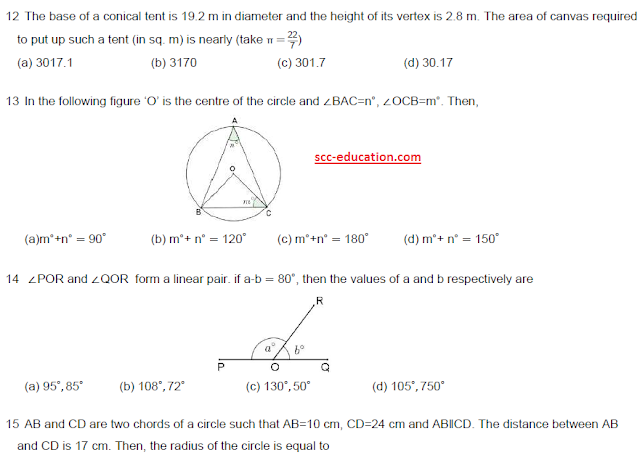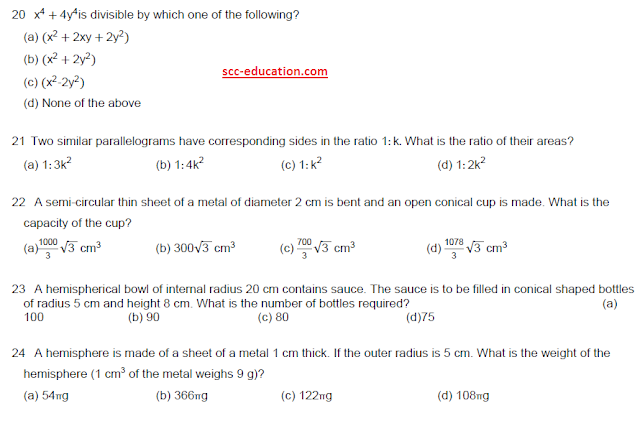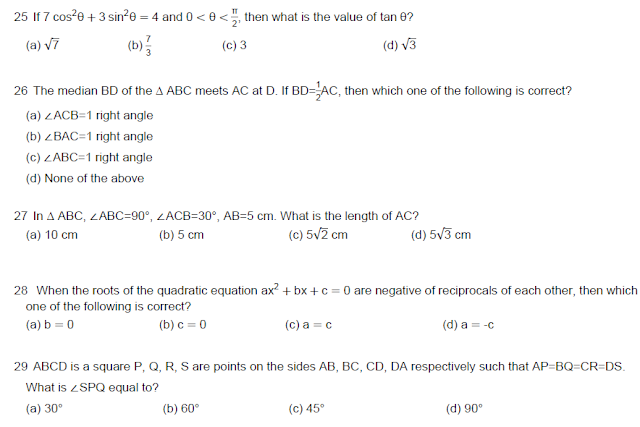# SCC Education

## Maths questions for entrance test,Delhi polytechnic combined entrance test ,Basic-trigonometry-identities
graphing-linear-inequalities solving
Introduction-of-co-ordinate-geometry
probability
materials-metals-and-non-metals
Trignometry-solved-questions
surface-areas-and-volumes
sequence
linear-equations